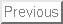1) (15 points) Let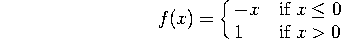a. Sketch the graph of f(x).

b. Find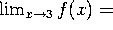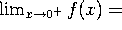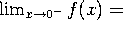c. Find the values of x where f is not continuous.

2) (25 points)

Compute the following limits: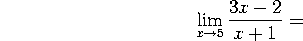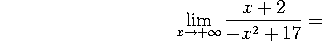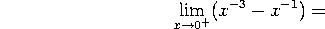3) (25 points)

Compute the derivative of the following functions. You don't need to simplify your answer: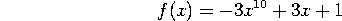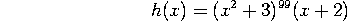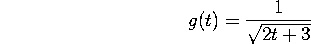4) (30 points)

Let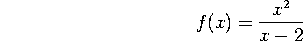a. Find the domain of f(x), the intercepts , vertical and horizontal asymptotes, if any.

b. Compute f'(x) and find the critical points of f.

c. Give the intervals where f is increasing and those where f is decreasing. Find all local maxima and all local minima.

d. Sketch the graph of f, using the previous information.

e. If f(x) for x;SPMgt;2 represents the cost function in thousands of dollars of producing x hundred wading pools, how many pools would you produce in order to minimize your cost? What would that minimal cost be?

5) (20 points)

Let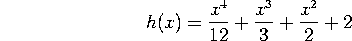a. Find the equation of the tangent line to the curve y=f(x) at x=0.

b. Compute f''(x). Give the intervals where f is concave upward and those where f is concave downward. Is there any inflection point for f?